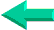This is an excerpt from Pierre Leclerc (www.excel-vba.com)

The 2 Most Important

Excel Functions AND
Formulas

SUMPRODUCT

AND

INDEX/MATCH

## Annex 6: Excel Spreadsheets Logical Functions (7)

There are 7 functions in the "Logical" category.

 Functions What it Does AND Returns TRUE if all its arguments are TRUE IF Specifies a logical test to perform NOT Reverses the logic of its argument OR Returns TRUE if any argument is TRUE FALSE Returns the logical value FALSE TRUE Returns the logical value TRUE New in Excel 2007 IFERROR Returns a specified value if the formula results in an error otherwise returns the result of the formula

SUMPRODUCT  ¦  INDEX/MATCH  ¦  SUBTOTAL  ¦  ISERROR  ¦  HLOOKUP  ¦  LOOKUP  ¦  VLOOKUP
AVERAGEIF  ¦  AVERAGEIFS ¦  COUNTIF  ¦  COUNTIFS  ¦  SUMIF  ¦  SUMIFS  ¦  IFERRORBack home